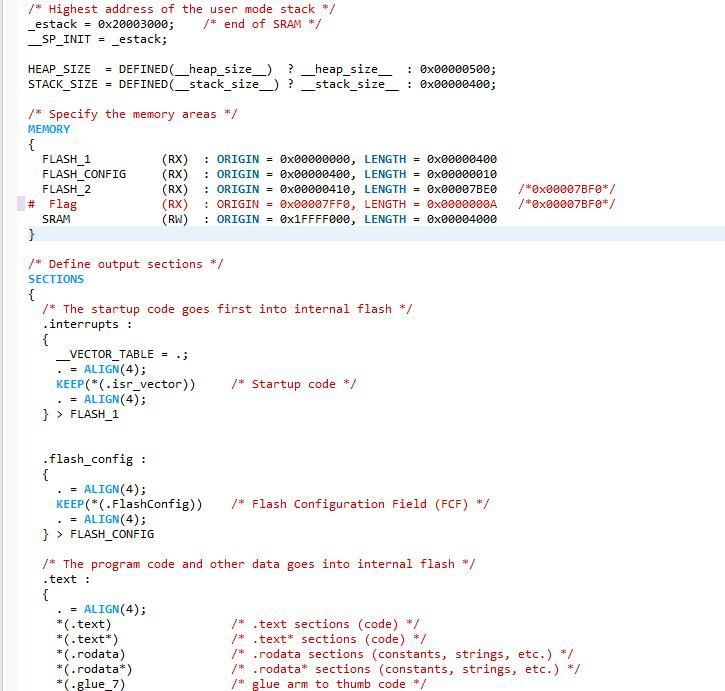## How to put a constant array into a specified flash memory?

cancel
Showing results for
Did you mean:
SOLVED

## How to put a constant array into a specified flash memory?

470 ViewsContributor II

Hello!

I want to put a constant array into a specified flash memory (address is 0x00007FF0)in S32 Design Studio for ARM.However,"const  char flag@0x00007FF0" doesn't work.

So,Should I modify the  Flash.ld?Tags (4)
1 Solution
188 ViewsNXP Employee

Hi,

/* Specify the memory areas */
MEMORY
{
/* Flash */
m_interrupts (RX) : ORIGIN = 0x00000000, LENGTH = 0x00000400
m_flash_config (RX) : ORIGIN = 0x00000400, LENGTH = 0x00000010
m_text (RX) : ORIGIN = 0x00000410, LENGTH = 0x00007BE0
m_mydata (RX) : ORIGIN = 0x00007FF0, LENGTH = 0x000000FF

/* SRAM_L */
m_data (RW) : ORIGIN = 0x1FFF8000, LENGTH = 0x00008000

/* SRAM_U */
m_data_2 (RW) : ORIGIN = 0x20000000, LENGTH = 0x00007000
}

/* Define output sections */
SECTIONS
{

.mydata :
{
KEEP(*(.mydata))
} > m_mydata

and define variable by using __attribute__ like this:

__attribute__ ((section(".mydata"))) const char flag[] = {"ABCDEFGHIJ"};

Jiri

2 Replies
189 ViewsNXP Employee

Hi,

/* Specify the memory areas */
MEMORY
{
/* Flash */
m_interrupts (RX) : ORIGIN = 0x00000000, LENGTH = 0x00000400
m_flash_config (RX) : ORIGIN = 0x00000400, LENGTH = 0x00000010
m_text (RX) : ORIGIN = 0x00000410, LENGTH = 0x00007BE0
m_mydata (RX) : ORIGIN = 0x00007FF0, LENGTH = 0x000000FF

/* SRAM_L */
m_data (RW) : ORIGIN = 0x1FFF8000, LENGTH = 0x00008000

/* SRAM_U */
m_data_2 (RW) : ORIGIN = 0x20000000, LENGTH = 0x00007000
}

/* Define output sections */
SECTIONS
{

.mydata :
{
KEEP(*(.mydata))
} > m_mydata

and define variable by using __attribute__ like this:

__attribute__ ((section(".mydata"))) const char flag[] = {"ABCDEFGHIJ"};

Jiri

188 ViewsContributor II

Hi，

Thank you very much for your help.

It's useful to add the "KEEP".I have solved the problem. :smileyhappy:

Best wishes

Mingming